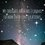# Differential coefficient of $x^2$ if $x$ is a positive integer

We all know that the differential coefficient of $x^2$ is $2x$. But if we write
$x^2 = \underbrace{x+ x+x+x+\cdots + x}_{x \text{ times}}$
On differentiating both sides with respect to $x$ we get: $d(x^2)/dx = \underbrace{1+ 1+1+1+\cdots + 1}_{x \text{ times}}$ = $x$
Why has the differential coefficient changed?

Similarly one can find differences in differential coefficients of $x^3$,$x^4$,$x$,etc. if $x$ is a positive integer.5 years ago

This discussion board is a place to discuss our Daily Challenges and the math and science related to those challenges. Explanations are more than just a solution — they should explain the steps and thinking strategies that you used to obtain the solution. Comments should further the discussion of math and science.

When posting on Brilliant:

• Use the emojis to react to an explanation, whether you're congratulating a job well done , or just really confused .
• Ask specific questions about the challenge or the steps in somebody's explanation. Well-posed questions can add a lot to the discussion, but posting "I don't understand!" doesn't help anyone.
• Try to contribute something new to the discussion, whether it is an extension, generalization or other idea related to the challenge.

MarkdownAppears as
*italics* or _italics_ italics
**bold** or __bold__ bold
- bulleted- list
• bulleted
• list
1. numbered2. list
1. numbered
2. list
Note: you must add a full line of space before and after lists for them to show up correctly
paragraph 1paragraph 2

paragraph 1

paragraph 2

[example link](https://brilliant.org)example link
> This is a quote
This is a quote
    # I indented these lines
# 4 spaces, and now they show
# up as a code block.

print "hello world"
# I indented these lines
# 4 spaces, and now they show
# up as a code block.

print "hello world"
MathAppears as
Remember to wrap math in $$ ... $$ or $ ... $ to ensure proper formatting.
2 \times 3 $2 \times 3$
2^{34} $2^{34}$
a_{i-1} $a_{i-1}$
\frac{2}{3} $\frac{2}{3}$
\sqrt{2} $\sqrt{2}$
\sum_{i=1}^3 $\sum_{i=1}^3$
\sin \theta $\sin \theta$
\boxed{123} $\boxed{123}$

Sort by:

First off, note that equation is valid only if $x$ is an integer. As the equation doesn't hold in any neighbourhood of a positive integer, you cannot differentiate the given equation on both sides!

Hence, the result of differentiating that equation is wrong!

That's right. It is just like differentiating xy wrt x and then substituting y=x.

- 5 years ago

Nice example.

- 5 years ago

Thank you I found my mistake.

- 5 years ago

If you look at it correctly, one way of interpreting is that the sum is only valid for for integer values. But another way of seeing it is that in writing the sum the numbers of times is x occurs is also a function in x, 'x times'. So it is possibly an implicit function of two variables. Like sum x y number of times. Now you can differentiate it wrt to x.

- 4 years, 11 months ago